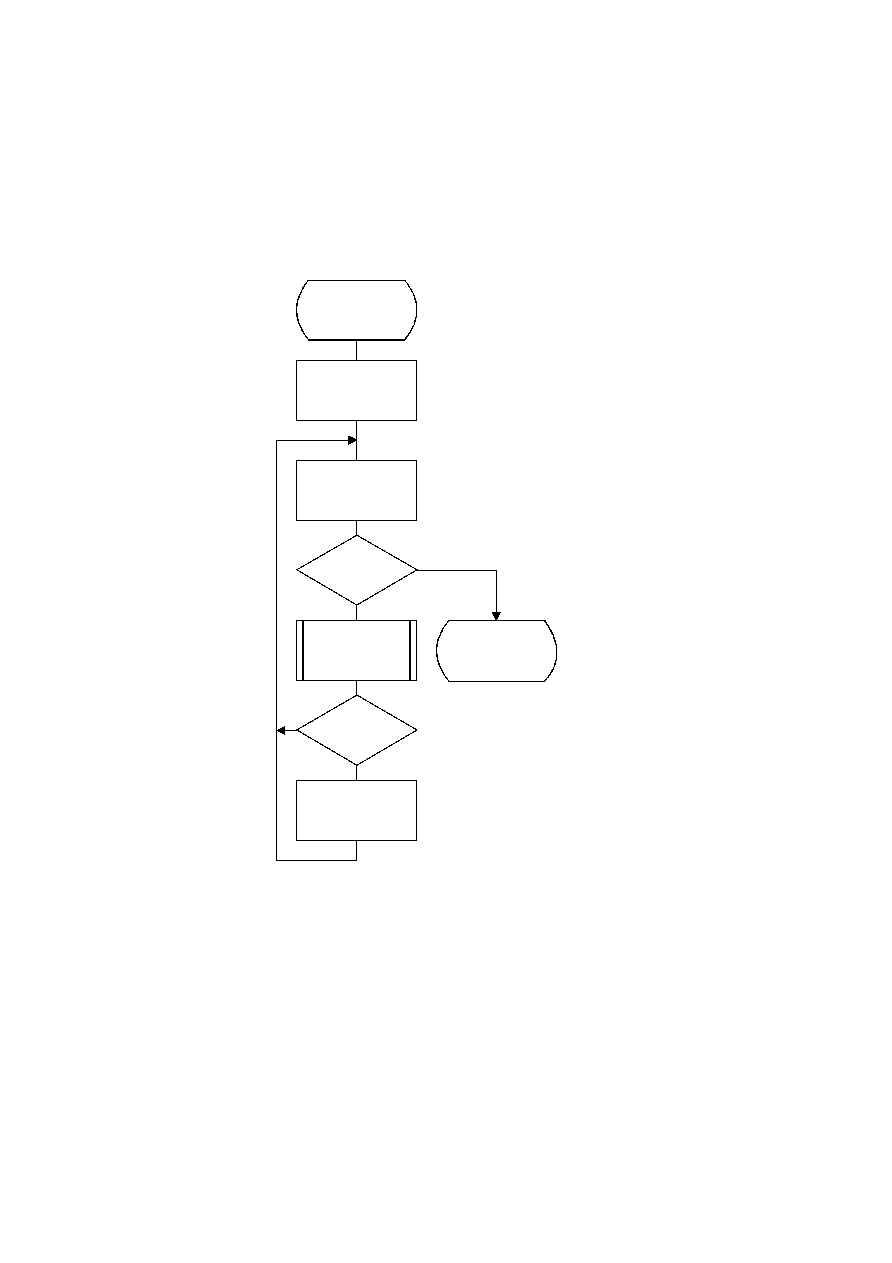ISO/IEC 10918-1 : 1993(E)
F.2.4.3.1.3
Decoding the exact value of the magnitude
After the magnitude category is decoded, the low order magnitude bits are decoded. These bits are decoded in order of
decreasing bit significance. The procedure is shown in Figure F.24.
The context-index S is set in Decode_log2_Sz.
TISO1560-93/d094
Decode_Sz_bits
S = S + 14
M = SRL M 1
M = 0
?
Yes
No
D = Decode(S)
Done
D = 0
?
Yes
No
Sz = M OR Sz
Figure F.24 ­ Decision sequence to decode the magnitude bit pattern
Figure F.24 [D94] = 16 cm = 625 %
F.2.4.4
Decoder restart
The RST
m
markers which are added to the compressed data between each restart interval have a two byte value which
cannot be generated by the coding procedures. These two byte sequences can be located without decoding, and can
therefore be used to resynchronize the decoder. RST
m
markers can therefore be used for error recovery.
CCITT Rec. T.81 (1992 E)
117